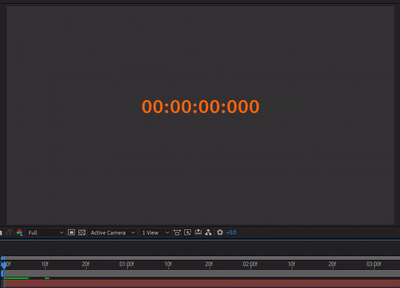### 《AE教學》用表達式做出計時器吧！表達式 / Timecod / Numbers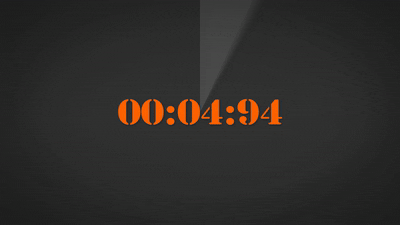## 1.TimecodeTimecode可以直接套用在任何圖層上，

Timecode能夠快速製作簡單的計時器，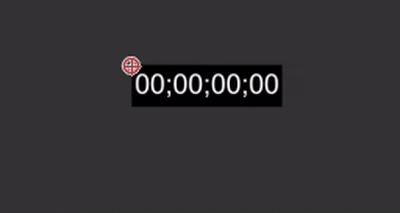## 2.NumbersNumbers相較Timecode就來的自由許多，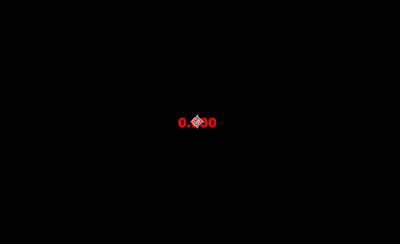## 3.表達式計時器countspeed = 1;
clockStart = 0;

function times(n) {
if (n<10) return "0" + n
else return "" + n
}

clockTime = clockStart + countspeed*(time – inPoint);

if (clockTime < 0) {
minus = "-";
clockTime = -clockTime;
} else {
minus = "";
}

t = Math.floor(clockTime);
min = Math.floor((t%3600)/60);
sec = Math.floor(t%60);
minus + times(min) + ":" + times(sec)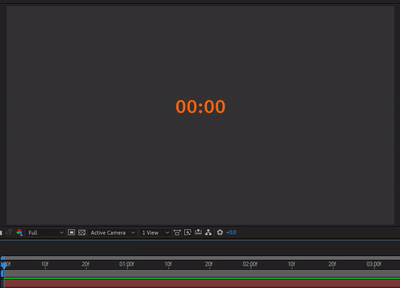t = Math.floor(clockTime);
min = Math.floor((t%3600)/60);
sec = Math.floor(t%60);
minus + times(min) + ":" + times(sec)

t = Math.floor(clockTime);
h = Math.floor(t/3600);
min = Math.floor((t%3600)/60);
sec = Math.floor(t%60);
ms = clockTime.toFixed(3).substr(-3);
minus + times(h) + ":" + times(min) + ":" + times(sec) + ":" + ms

minus + times(h) + ":" + times(min) + ":" + times(sec) + ":" + msms = clockTime.toFixed(3).substr(-3);

ms = clockTime.toFixed(2).substr(-2);